#####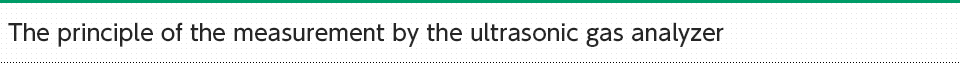####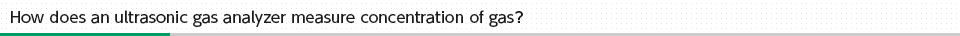Although the speed of sound is generally known as about 340 m/s, this is the speed of sound in our atmosphere (i.e., approx.21% oxygen, Approx.79% nitrogen and at the temperature of 25℃).
If composition and temperature of gas changes, the speed of sound will also change.

The ultrasonic gas analyzer uses this characteristics.

The sensor for transmission and the sensor for reception are placed facing at each other and the sensor for transmission sends ultrasonic waves.
Ultrasonic waves pass through the measured gas and the time to reach the sensor for reception is measured.####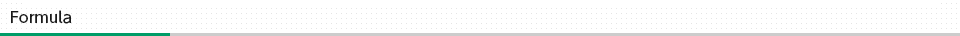Since the distance between sensors is known, the speed of sound can be calculated.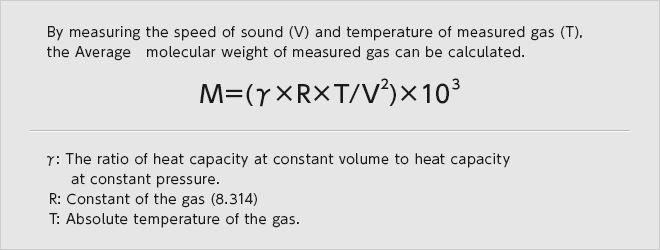If the Average molecular weight of measured gas is known, each concentration of two known gases is found.
The speed of sound becomes 339.919 m/s under the environment of 50% oxygen, 50% nitrogen, and temperature of 25℃.
When The speed of sound is assigned to the formula, the average molecular weight can be calculated as approx. 30.Since the difference of molecular weights between oxygen and nitrogen is approximately 4.
28 of the molecular weight of nitrogen is subtracted from 30 of the average molecular weight, and its answer is divided by 4, so the oxygen concentration will be calculated as approximately 50%.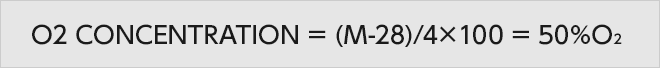####Although we mentioned the oxygen concentration measurement in nitrogen as the example in the above explanation, The ultrasonic gas analyzer can theoretically measure all mixture of two gases. The measurable minimum digit improves more if the difference of the molecular weight between two gases is larger.
As for each speed of sound at the gas temperature of 25℃ are 329.23m/s in 100% oxygen , 351.88m/s in 100% nitrogen, and 1316.93 m/s in 100 % hydrogen.
When the distance between sensors is 10cm, the arrival times interval of oxygen- nitrogen mixed gas is approximately 20μS.
If the CPU can scan only 0.2μS, only 100 counts can be measured, so the measurable minimum digit would be 1%.
If in hydrogen-nitrogen mixed gas, the arrival times interval is approximately 208μS. The same CPU can measure approximately 1000 counts and the measurable minimum digit would be 0.1%.
If the CPU can scan 0.02μS, approximately 1000 counts can be measured, so the measurable minimum digit would be 0.1% even in oxygen-nitrogen mixed gas.
Moreover, if the distance between sensors is extended from 10cm to 20cm,
the arrival time interval would also be extended from 20μS to 40μS,
so the measurable minimum digit would become twice as small even by the CPU which can scan only 0.2μS.
Thus, there are still more elements which improve performances of the ultrasonic gas analyzer and the wide use in future is expected.

Oxygen Nitrogen Hydrogen
Molecular weight(g/mol) 32 28 2
The speed of sound(m/s) 329.23 351.88 1316.93
Arrival times(μS) 303.95 284.19 75.93
Arrival times interval(μS) 19.76 208.26
Measurable minimum digit Approx.1% Approx.0.1%
Approx.0.1% Approx.0.01%

####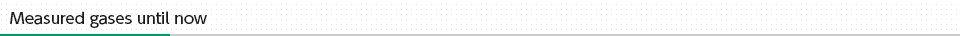Oxygen(O2), Nitrogen(N2), Hydrogen(H2), Carbon dioxide(CO2), Helium(He), Argon(Ar), Xenon(Xe), Krypton(Kr), Neon(Ne), Sulfur hexafluoride(SF6), Carbon tetrafluoride(CF4), Nitrous oxide（Laughing gas,N2O）